Question

# For a continuous time linear time-invariant system, the input-output relation is the following (x(t) the input, y(t) the...

For a continuous time linear time-invariant system, the
input-output relation is the following (x(t) the input, y(t) the
output):

, where h(t) is the impulse response function of the system.
Please explain why a signal like e/“* is always an eigenvector of
this linear map for any w. Also, if ¥(w),X(w),and H(w) are the
Fourier transforms of y(t),x(t),and h(t), respectively. Please
derive in detail the relation between Y(w),X(w),and H(w),
which means to reproduce the proof of the basic convolution
property of Fourier transform.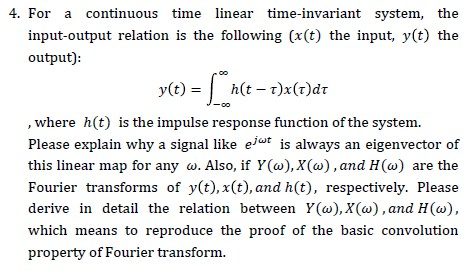4. For a continuous time linear time-invariant system, the input-output relation is the following (x(t) the input, y(t) the output) y(t)h(t-T)x (r) d , where h(t) is the impulse response function of the system Please explain why a signal like eir is always an eigenvector of this linear map for any o. Also, if Y(a),X(w), and H(w) are the Fourier transforms of y(t), x(t), and h(t), respectively. Please derive in detail the relation between Y(w), X(w) , and H(w) which means to reproduce the proof of the basic convolution property of Fourier transform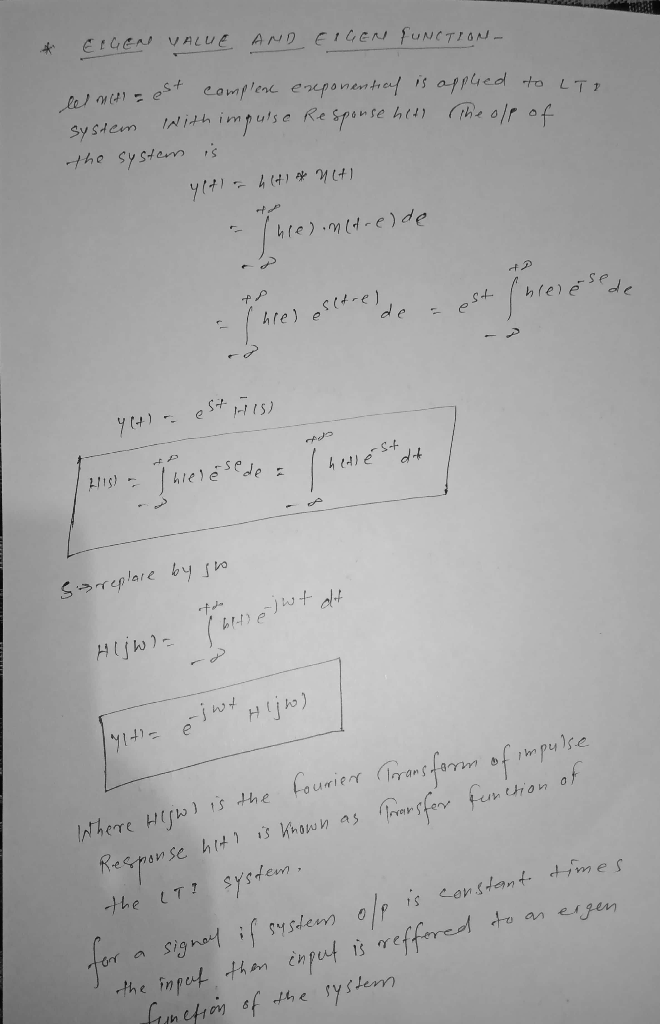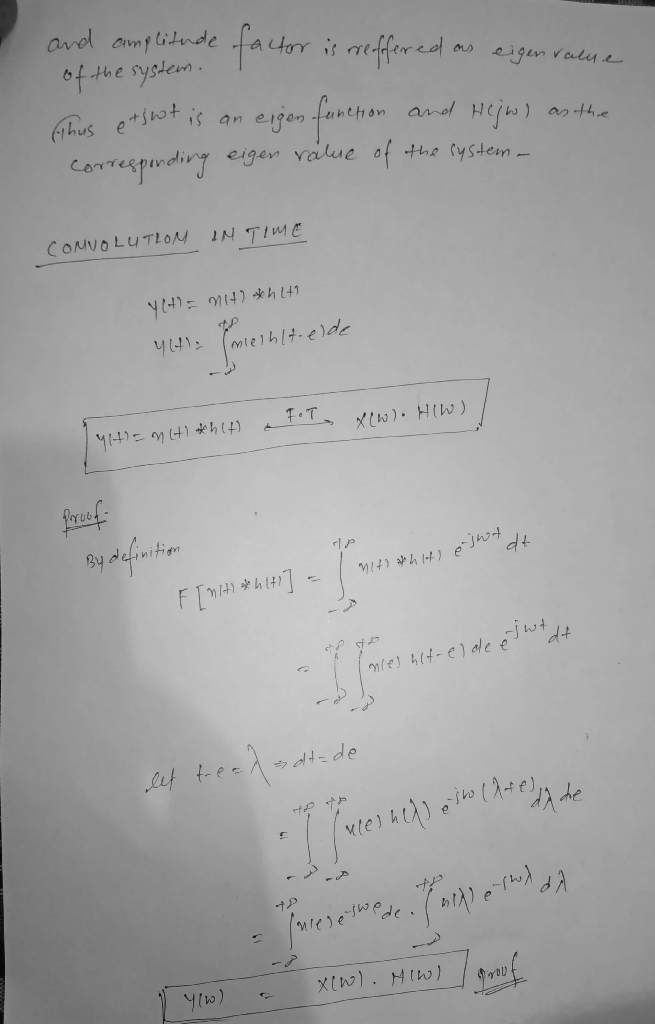#### Earn Coins

Coins can be redeemed for fabulous gifts.

Similar Homework Help Questions
• ### Consider a causal, linear and time-invariant system of continuous time, with an input-output relation that obeys...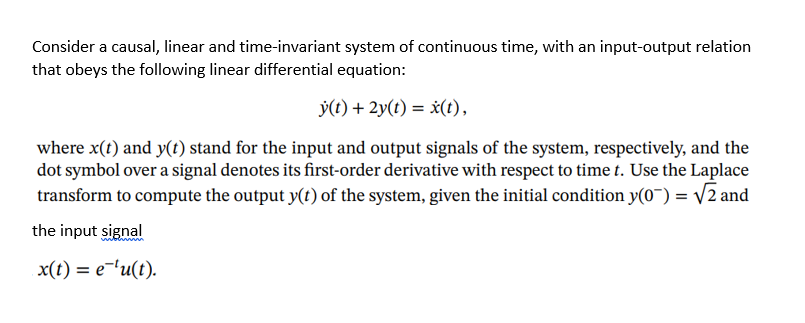Consider a causal, linear and time-invariant system of continuous time, with an input-output relation that obeys the following linear differential equation: y(t) + 2y(t) = x(t), where x(t) and y(t) stand for the input and output signals of the system, respectively, and the dot symbol over a signal denotes its first-order derivative with respect to time t. Use the Laplace transform to compute the output y(t) of the system, given the initial condition y(0-) = V2 and the input signal...

• ### 2. Let y(t)(e')u(t) represent the output of a causal, linear and time-invariant continuous-time system with unit...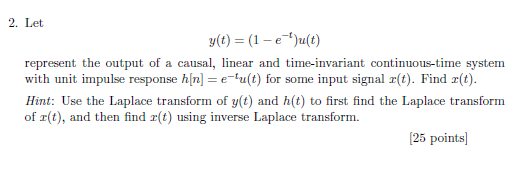2. Let y(t)(e')u(t) represent the output of a causal, linear and time-invariant continuous-time system with unit impulse response h[nu(t) for some input signal z(t). Find r(t) Hint: Use the Laplace transform of y(t) and h(t) to first find the Laplace transform of r(t), and then find r(t) using inverse Laplace transform. 25 points

• ### 4. Let S be a linear, time-invariant, and causal system whose input x(t) and corresponding output...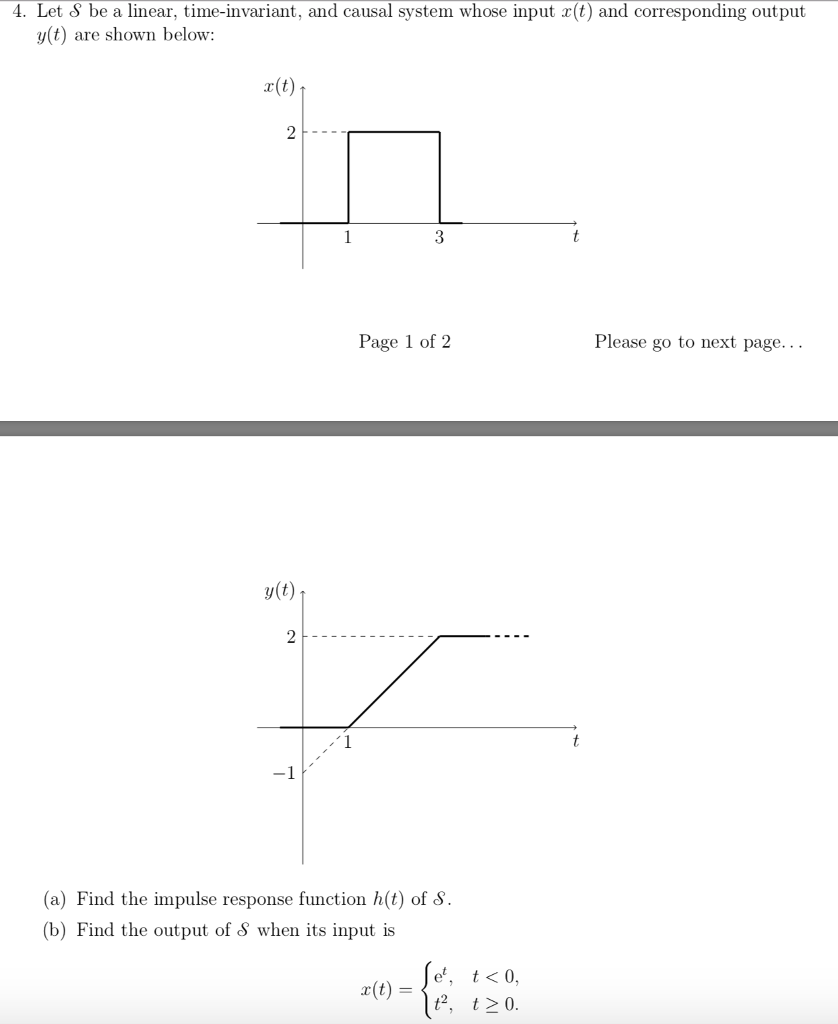4. Let S be a linear, time-invariant, and causal system whose input x(t) and corresponding output y(t) are shown below: r(t) Page 1 of 2 Please go to next page... y(t) ? (a) Find the impulse response function h(t) of ? (b) Find the output of S when its input is e*, t<0, t2, t20

• ### solve all 22. The input-output relationship for a linear, time-invariant system is described by differential equation...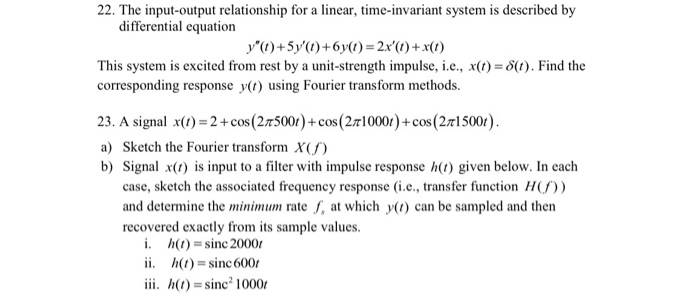solve all 22. The input-output relationship for a linear, time-invariant system is described by differential equation y") +5y'()+6y(1)=2x'()+x(1) This system is excited from rest by a unit-strength impulse, i.e., X(t) = 8(t). Find the corresponding response y(t) using Fourier transform methods. 23. A signal x(1) = 2 + cos (215001)+cos (210001)+cos (2.15001). a) Sketch the Fourier transform X b) Signal x() is input to a filter with impulse response (1) given below. In each case, sketch the associated frequency response...

• ### From homework section of CD: Continuous-time convolution Consider the linear time-invariant system shown below. 7ylt) (t)...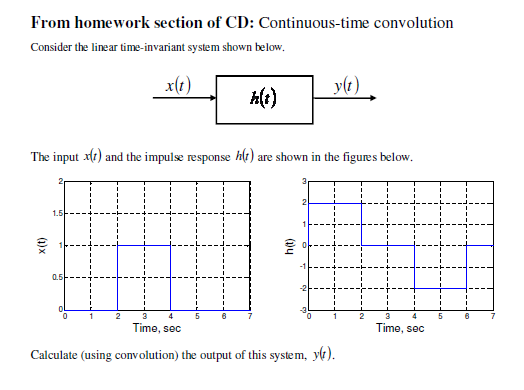From homework section of CD: Continuous-time convolution Consider the linear time-invariant system shown below. 7ylt) (t) The input alt) and the impulse response h(t) are shown in the figures below. ht) Time, sec Time, sec Calculate (using convolution) the output of this system, yo).

• ### QUESTION 2 (20 MARKS) (a) A continuous time signal x(t) = 3e2tu(-t) is an input to...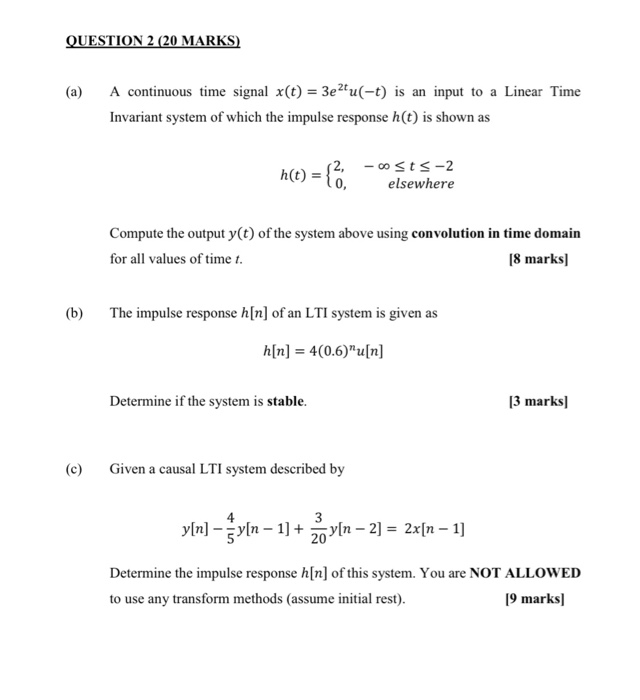QUESTION 2 (20 MARKS) (a) A continuous time signal x(t) = 3e2tu(-t) is an input to a Linear Time Invariant system of which the impulse response h(t) is shown as h(t) = { .. 12, -osts-2 elsewhere Compute the output y(t) of the system above using convolution in time domain for all values of time t. [8 marks) (b) The impulse response h[n] of an LTI system is given as a[n] = 4(0.6)”u[n] Determine if the system is stable. [3...

• ### Consider a first-order system with input x(t) and output y(t). Let the time constant be the...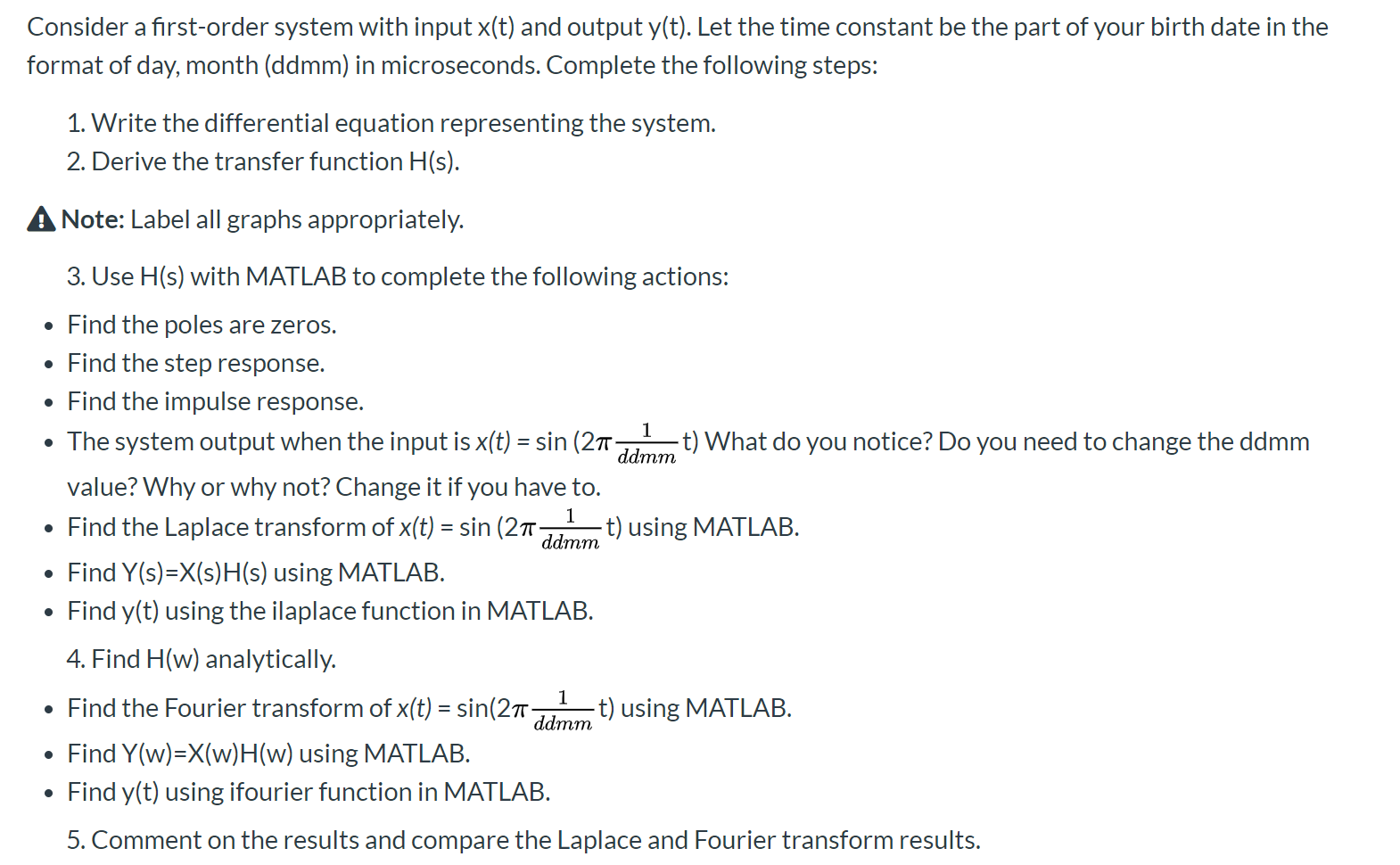Consider a first-order system with input x(t) and output y(t). Let the time constant be the part of your birth date in the format of day, month (ddmm) in microseconds. Complete the following steps: 1. Write the differential equation representing the system. 2. Derive the transfer function H(s). A Note: Label all graphs appropriately. ddmm 3. Use H(s) with MATLAB to complete the following actions: • Find the poles are zeros. • Find the step response. • Find the impulse...

• ### If of the input signal X(t) and output signal yl to a continuous - time linear...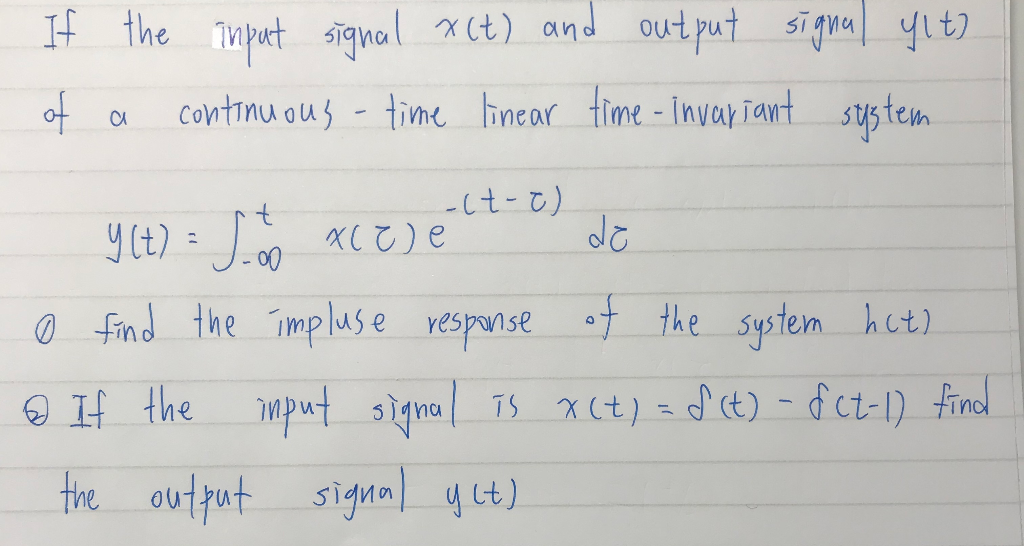If of the input signal X(t) and output signal yl to a continuous - time linear time - invariant system - Y(t) = 5 to xc ej elt- el de o find the impluse response of the system hat) o If the input signal is x (t) = f(t) - f (t-1) find the output signal y ct) o Find the impulse response of the system hit) @ If the input signal Is x (t) = f(t)-f(t-1) find the -...

• ### 6. (15) Consider the following causal linear time-invariant (LTT) discrete-time filter with input in and output...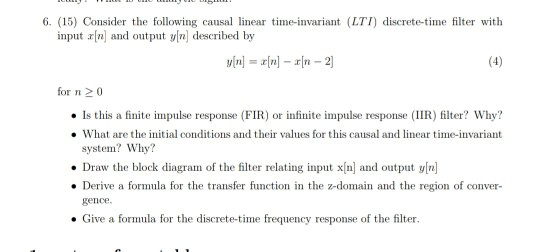6. (15) Consider the following causal linear time-invariant (LTT) discrete-time filter with input in and output yn described by y[n] = x[n] – rn - 2 for n 20 . Is this a finite impulse response (FIR) or infinite impulse response (IIR) filter? Why? • What are the initial conditions and their values for this causal and linear time-invariant system? Why? • Draw the block diagram of the filter relating input x[n) and output y[n] • Derive a formula for...

• ### Problem 4. Linear Time-Invariant System.s A linear system has the block diagram y(t) z(t) →| Delay...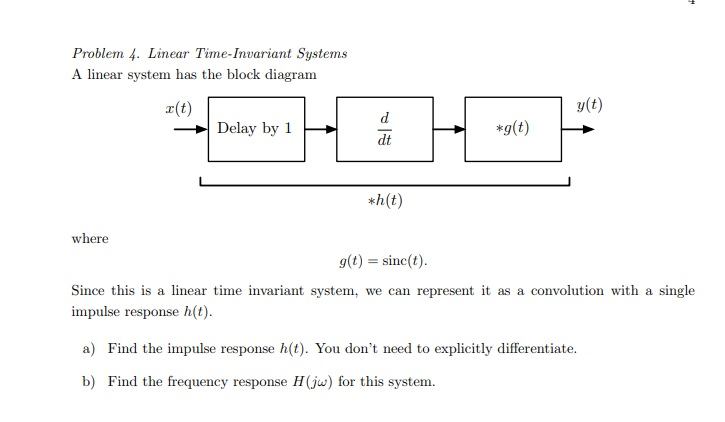Problem 4. Linear Time-Invariant System.s A linear system has the block diagram y(t) z(t) →| Delay by 1 dt *h(t) where g(t) sinc(t Since this is a linear time invariant system, we can represent it as a convolution with a single impulse response h(t) a) Find the impulse response h(t). You don't need to explicitly differentiate. b) Find the frequency response H(j for this system.